Question

# Draw the Lewis structures of the molecules below and use them to answer the following questions:...

Draw the Lewis structures of the molecules below and use them to answer the following questions: I. BH3 II. NO2 III. SF6 IV. O3 V. PCl5

How many of the molecules have no dipole moment?

A. 2

B. 3

C. 1

D. 4

E. They are all polar.

We need at least 10 more requests to produce the answer.

0 / 10 have requested this problem solution

The more requests, the faster the answer.

All students who have requested the answer will be notified once they are available.

#### Earn Coins

Coins can be redeemed for fabulous gifts.

Similar Homework Help Questions
• ### QUESTION 14 Draw the Lewis structures of the molecules below and use them to answer the...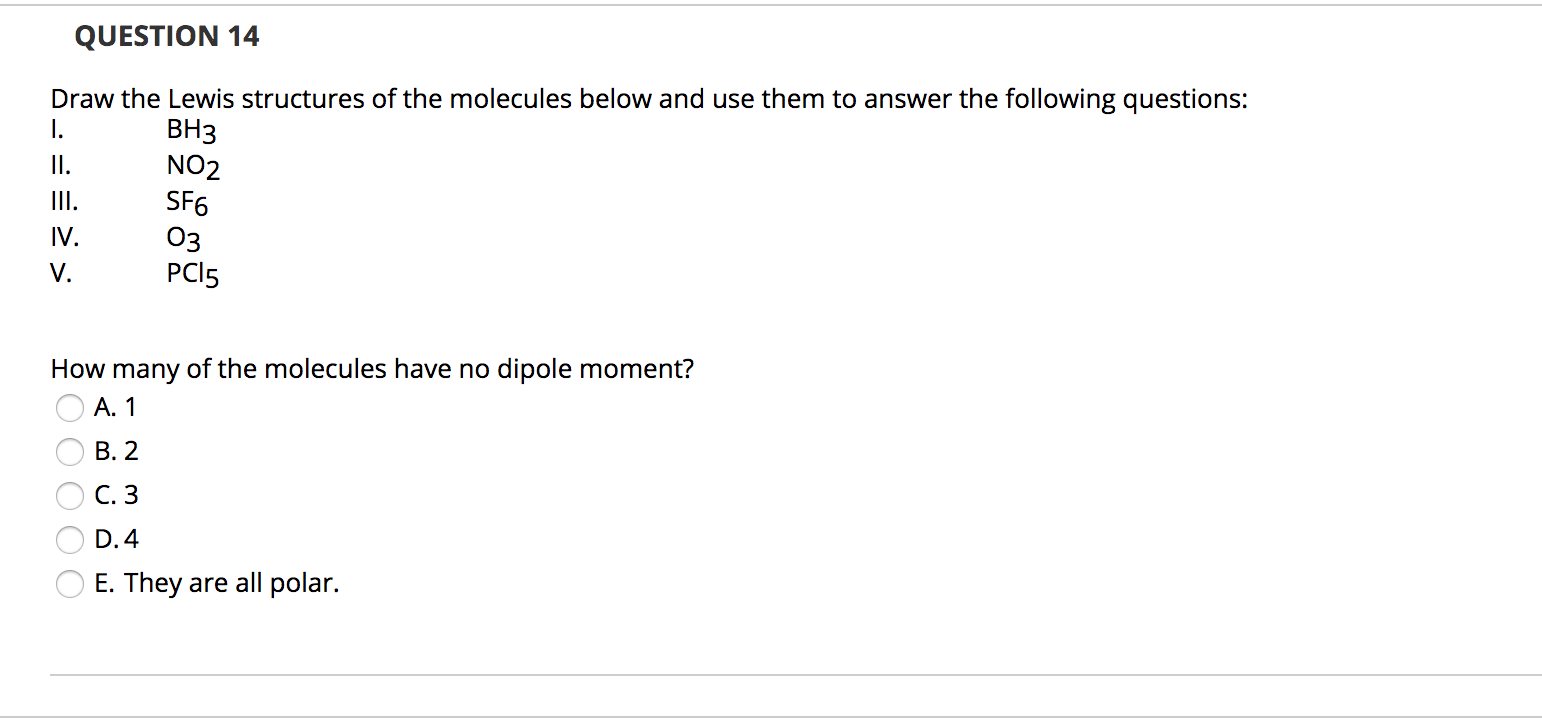QUESTION 14 Draw the Lewis structures of the molecules below and use them to answer the following questions: BH3 III. NO2 SF6 03 PC15 IV. How many of the molecules have no dipole moment? O A. 1 O B.2 O C.3 O D.4 O E. They are all polar.

• ### Draw the Lewis structures of the molecules below (note: first atom is the central atom) and...

Draw the Lewis structures of the molecules below (note: first atom is the central atom) and use them to answer the following questions: I. BH3 II. SeF6 III. O3 IV. NO2 V. POCl3 Which molecule(s) obey(s) the Octet rule? A. IV and V B.III and IV C.I, III and IV D.III only E. IV only (Continuing from the previous question) How many of the molecules are planar (i.e., all atoms lie in the same plane)? One Two Three Four Five

• ### (9) For each pair of molecules or ions below (i) Draw the Lewis dot structures. (If...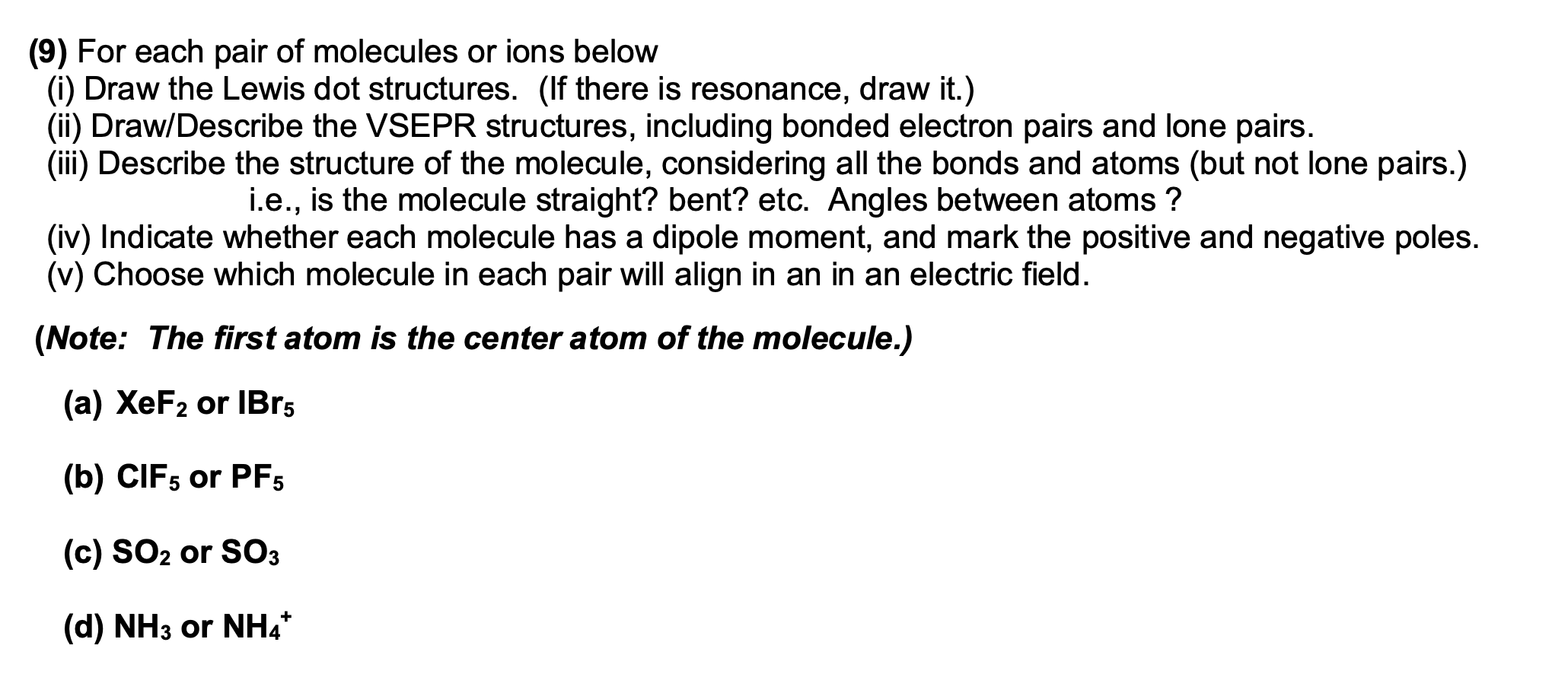(9) For each pair of molecules or ions below (i) Draw the Lewis dot structures. (If there is resonance, draw it.) (ii) Draw/Describe the VSEPR structures, including bonded electron pairs and lone pairs. (iii) Describe the structure of the molecule, considering all the bonds and atoms (but not lone pairs.) i.e., is the molecule straight? bent? etc. Angles between atoms? (iv) Indicate whether each molecule has a dipole moment, and mark the positive and negative poles. (v) Choose which molecule...

• ### 3. Of the molecules listed, which does not have a dipole moment? (Hint: Draw them out as Lewis structures with prop...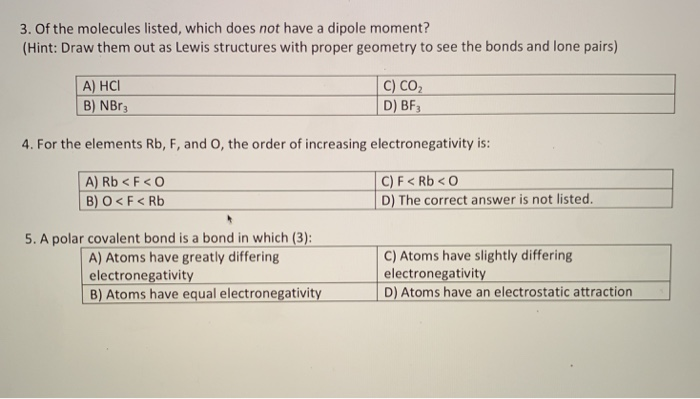3. Of the molecules listed, which does not have a dipole moment? (Hint: Draw them out as Lewis structures with proper geometry to see the bonds and lone pairs) C) CO D) BF3 A) HC B) NBR3 4. For the elements Rb, F, and O, the order of increasing electronegativity is: A) Rb < F<O B) O<F Rb C)F< Rb<O D) The correct answer is not listed. 5.A polar covalent bond is a bond in which (3): C) Atoms have...

• ### 5.) Draw Lewis dot structures for the following molecules below. Next, draw the three dimensional structure for each...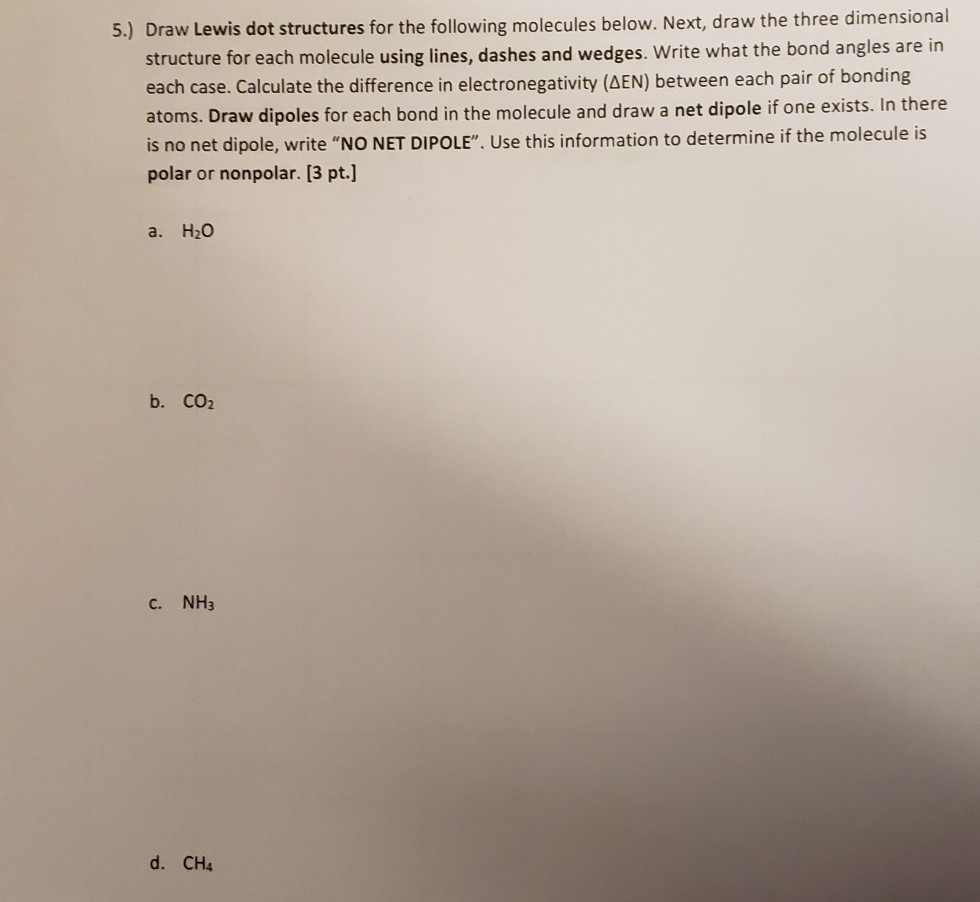5.) Draw Lewis dot structures for the following molecules below. Next, draw the three dimensional structure for each molecule using lines, dashes and wedges. Write what the bond angles are in each case. Calculate the difference in electronegativity (AEN) between each pair of bonding atoms. Draw dipoles for each bond in the molecule and draw a net dipole if one exists. In there is no net dipole, write "NO NET DIPOLE". Use this information to determine if the molecule is...

• ### 3. Draw resonance structures for the following molecules. favorable structure, if any Calculate formal charge for...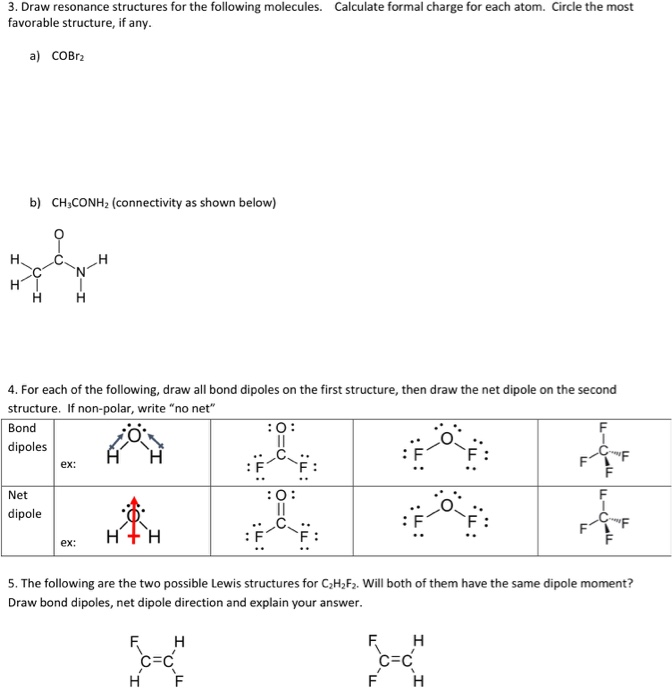3. Draw resonance structures for the following molecules. favorable structure, if any Calculate formal charge for each atom. Circle the most a) сОвг» b) CH CONH2 (connectivity as shown below) Н. H Н 4. For each of the following, draw all bond dipoles on the first structure, then draw the net dipole on the second structure. If non-polar, write "no net" Bond :O: dipoles ex: : F Net O: dipole F F H+H ex: 5. The following are the two...

• ### Draw the resonance structures for O3. Which one of the following molecules is polar: CO2 or...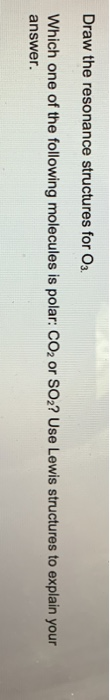Draw the resonance structures for O3. Which one of the following molecules is polar: CO2 or SO2? Use Lewis structures to explain your answer.

• ### Draw the best Lewis dot structures for the 6 molecules below, and answer the questions that...

Draw the best Lewis dot structures for the 6 molecules below, and answer the questions that follow. N2H2 BF3 I3+1 CN-1 SH4 CH3P Which compound(s) have an atom with more than 8 electrons? Which compound(s) have an atom with only 6 electrons?

• ### For each of the following molecules, (1) Draw the individual bond dipole moments. (2) If it...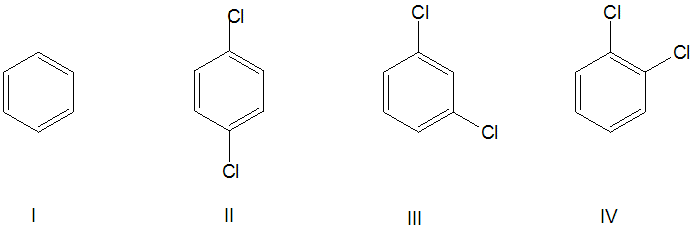For each of the following molecules, (1) Draw the individual bond dipole moments. (2) If it is polar, show the net dipole moment as well. (3) Rank the molecules in order of increasing polarity. Ū 0 ) CI - II III IV

• ### Draw Lewis structures for the following molecules and predict their molecular shapes: h. PF5 i. BrF3...

Draw Lewis structures for the following molecules and predict their molecular shapes: h. PF5 i. BrF3 j. SF4 k. IF2 - l. BrF5 m. ICl4 - n. SF6